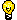Click to Chat

1800-1023-196

+91-120-4616500

CART 0

• 0

MY CART (5)

Use Coupon: CART20 and get 20% off on all online Study Material

ITEM
DETAILS
MRP
DISCOUNT
FINAL PRICE
Total Price: Rs.

There are no items in this cart.
Continue Shopping```Sum of Binomial CoefficientsPutting x = 1 in the expansion (1+x)n = nC0 + nC1 x + nC2 x2 +...+ nCx xn, we get,

2n = nC0 + nC1 x + nC2 +...+ nCn.

We kept x = 1, and got the desired result i.e. ∑nr=0  Cr = 2n.

Note:  This one is very simple illustration of how we put some value of x and get the solution of the problem. It is very important how judiciously you exploit this property of binomial expansion.

Illustration:

Find the value of C0 + C2 + C4 +... in the expansion of (1+x)n.

Solution:

We have,

(1 + x)n = nC0 + nC1 x + nC2 x2 +... nCn xn.

Now put x = -x; (1-x)n = nC0 - nC1 x + nC2 x2 -...+ (-1)n nCn xn.

Now, adding both expansions, we get,

(1 + x)n + (1-x)n = 2[nC0 + nC2 x2 + nC4 x4 +.........]

Put    x = 1

=>     (2n+0)/2 = C0 + C2 + C4 +......

or     C0 + C2 + C4 +......= 2n-1

We have found the sum of binomial coefficients. But if these coefficients are multiplied by some factors can we find the sum for such expressions?

Yes, we can often find it by creatively applying what we have learnt. Let us see how differentiation and integration are useful in these situations.

Suppose we want to calculate the value of

∑nr=0  Cr i.e. 0.C0 + 1 C1 + 2C2 +...+ n nCn

If we take a close look to the sum to be found, we find that coefficients are multiplied with respective powers of x.

If we want to multiply the coefficient of x by its power differentiation is of help. Hence differentiate both sides of

(1 + x)n = nC0 + nC1 x + nC2 x2 + nC3 x3 +...+ nCn xn, with respect to x we get

n(1 + x)n-1 = 1 C1 x1-1 + 2 C2 x2-1 +...+ n Cn xn-1

Put x = 1, we get, n 2n-1 = + 1 C1 + 2 C2 +...+ n Cn.

Or,      ∑nr=0     r  Cr = n 2n-1, which is the answer.

Now, think how to find the following sum

C0/1 + C1/2 + C2/3 +......+ Cn/n+1 = ?

In this sum coefficients are divided by the respective power of x + 1. This expression can be achieved by Integrating the expansion of (1 + x)n under proper limits.Important:  It has said earlier in this chapter that we should use and exploit the property that x can take any value in the expansion of (1 + x)n.

Now, let us try to find the value of

C0 - C2 + C4-C6 +.........

Analysing the above, expression, we find that C0, C2, C4 are all coefficients of even powers of x.

Had it been like C0 + C2 + C4 +...... we could have evaluated simply by the method described earlier or had it been like C0-C1 + C2-C3 +... we could have put x = -1 in the expansion of (1 + x)n and find the sum.

But here the case is different. The expression consists of coefficients of only even powers. In such cases when there is alternative sign charge for the coefficient, of same nature of powers (even or odd) use of I (iota) comes to our rescue.

(1 + x)n = C0 + C1 x + C2 x2 +......

(1 - x)n = C0 - C1 x + C2 x2 +......

By adding   (1 + x)n + (1 - x)n = 2[C0 + C2 x2 + C4 x4 +......].

Clearly use of √-1 (iota) will generate the given expression.

((1+i)n + (1-i)n)/2 ] [C0 - C2 + C4 + ......]

The platform at askIITians is the only group of IITians which provide you free online courses and a good professional advice for IIT JEE, AIEEE and other Engineering Examination preparation. You can also visit askIITians.com to study topics pertaining to the IIT JEE and AIEEE syllabus for free.

To read more, Buy study materials of Binomial Theorem comprising study notes, revision notes, video lectures, previous year solved questions etc. Also browse for more study materials on Mathematics here.
```### Course Features

• 731 Video Lectures
• Revision Notes
• Previous Year Papers
• Mind Map
• Study Planner
• NCERT Solutions
• Discussion Forum
• Test paper with Video Solution